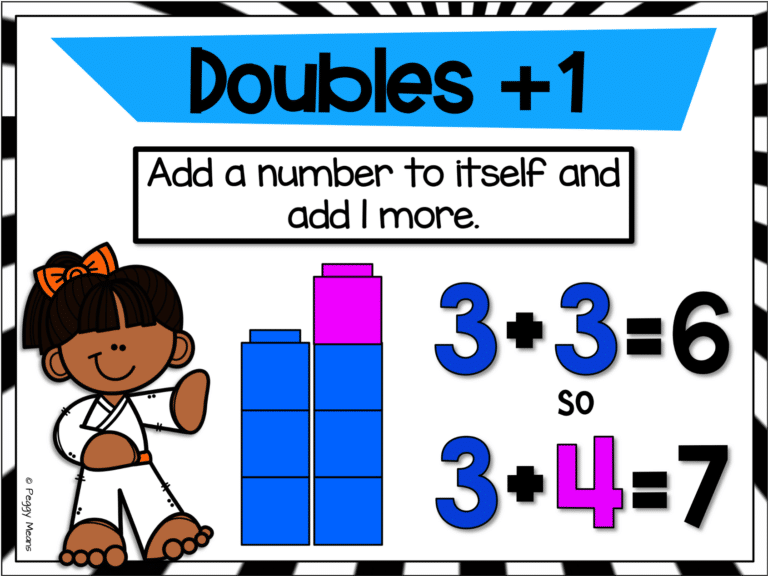## What is Math Fact Fluency?

Math fact fluency is the ability to quickly recall
the answers to basic math facts automatically – without hesitation.

## Why is Math Fact Fluency Important?

#### So WHY do we need to help our students become fluent in basic math facts?

Quite simply, a lack of fluency in basic math facts will significantly hinder a child's subsequent progress in problem-solving, algebra, and higher order math concepts.

For these reasons, and probably a zillion more, the Common Core Standards require us to insure that our students are fluent in their math facts.

Math fact automaticity will affect performance in later elementary grades as students have longer and more complicated computations to complete.

At this stage, if a student does not have his/her math facts committed to memory, he/she will spend a lot of time and energy figuring out smaller calculations and risk not having time (or energy) to complete the assignment.

Their performance in math class is not the only academic area that may be negatively affected.  Other subjects such as science and geography may be hindered.

## How to Increase Math Fact Fluency

Math fact fluency is developed through lots of practice with effective tools and strategies.

I have found, and research confirms that Derived Fact Strategies (DFS) are highly effective in increasing math fact fluency.

Derived facts are math facts that are derived from known facts.

For example, if we know the doubles fact, 3+3=6, then we can derive the answer to 3+4 by using the 3+3 fact and adding 1 to it.

So a derived fact strategy is the mental process of deriving a new fact from a known fact.  We can see in the picture below how we use the ‘Doubles +1' strategy to derive an sum by using a known sum.These strategies are also called ‘mental math strategies‘ and ‘thinking strategies'.  They all refer to the cognitive process of deriving new understanding by using known information – higher-level thinking!

## Are Derived Fact Strategies Aligned to Common Core Standards?

Yes, they are aligned to the Common Core Standards:

###### Add and subtract within 20:
CCSS.MATH.CONTENT.1.OA.C.5
Relate counting to addition and subtraction (e.g., by counting on 2 to add 2).

CCSS.MATH.CONTENT.1.OA.C.6
Add and subtract within 20, demonstrating fluency for addition and subtraction within 10. Use strategies such as counting on; making ten (e.g., 8 + 6 = 8 + 2 + 4 = 10 + 4 = 14); decomposing a number leading to a ten (e.g., 13 – 4 = 13 – 3 – 1 = 10 – 1 = 9); using the relationship between addition and subtraction (e.g., knowing that 8 + 4 = 12, one knows 12 – 8 = 4); and creating equivalent but easier or known sums (e.g., adding 6 + 7 by creating the known equivalent 6 + 6 + 1 = 12 + 1 = 13).

CCSS.MATH.CONTENT.2.OA.B.2
Fluently add and subtract within 20 using mental strategies.2 By end of Grade 2, know from memory all sums of two one-digit numbers.

I have seen students transform from discouraged to confident learners as they master these strategies!

And they start to enjoy math!

I have also seen the flip side where teachers are clinging to timed tests traditional flashcards and pushing the responsibility of math fact fluency onto the parents (much to their frustration) –

And that creates frustrated students – even tears – and negative experiences in math for years.

Knowing that lots of practice opportunities are crucial for students to become ‘automatic' in their recall of basic facts, I have invested hundreds of hours creating engaging resources to provide students (and teachers) with engaging and effective activities that make practice fun!

Games have always been a hit with students and teachers! Learning games provide a fun, non-threatening opportunity for students to get the needed practice to become fluent in their math facts.

I have four FREE games for you that provide repeated experience with the DFS (derived fact strategies):  Plus 0.  Included with the games is a strategy poster so that you can teach and review the strategies with your students.

Just fill out the form below, and I will send it to your inbox!

Your students will be engaged and having fun.  You will not have any papers to grade!  Sounds like a WIN-WIN!

#### Check out more strategy-based

The following math program provides additional resources for you to

• Teach
• Motivate
• Provide Independent Practice
• Access student mastery of mental math strategies.

Everything you need to set up an effective math fact fluency program in your classroom!

If you have not tried teaching the Derived Fact Strategies (DFS) with your students,

I hope you will!

If you do teach them – keep on, keeping on!

#### WelcomeMy name is Peggy Means. I am a child of God and a retired elementary teacher. I loved teaching and creating engaging resources for my students. Now, I love sharing my resources and ideas with busy teachers around the world.

Need Some Freebies?